# Macroeconomics Study Set 62

## Quiz 9 : Demand-Side Equilibrium: Unemployment or InflationStudy FlashcardsLooking for Economics Homework Help?

## Quiz 9 :Demand-Side Equilibrium: Unemployment or Inflation

Question TypeFor about 30 years now, imports have consistently exceeded exports in the U.S. economy. Many people consider this imbalance to be a major problem. Does this chapter give you any hints about why (You may want to discuss this issue with your instructor. You will learn more about it in later chapters.)
Free
Essay

If the imports of an economy consistently exceed the exports of an economy, then the net exports will be negative.
Negative net exports will push down the equilibrium level of GDP
Based on the discussion in the chapter, increase in net exports will have a multiplier effect on GDP. It means a small increase in net exports will cause an increase in GDP at a higher rate. On the other hand, a small fall in net exports will cause the GDP to fall at a higher rate.
Thereby, the multiplier effect of negative net exports will push the equilibrium level of GDP down.

Tags
Choose question tagExplain the basic logic behind the multiplier in words. Why does it require b, the marginal propensity to consume, to be between 0 anD₁
Free
Essay

Definition of Multiplier
The multiplier effect explains the effect of change in any component of GDP on GDP. The consumption multiplier effect depends on the marginal propensity to consume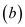, which lies within the range of zero to one.
Marginal propensity to consume is the additional units of consumption made to the total consumption in response to change in disposable income.
Reason behind range of b is zero to one
Marginal propensity to consume lies in the range of zero to one because income can be either consumed or saved; if whole income is consumed, then b will be₁ and multiplier effect will be infinitive. On the other hand, if whole income is saved, then consumption is zero; hence, the multiplier effect of consumption will be zero. It means the b coefficient will be zero.
Since the sum of consumption and saving cannot exceed the total income, the value of b coefficient lies within 0 to 1.

Tags
Choose question tagLook back at the income-expenditure diagram in Figure 3 and explain why some level of real GDP other than $6,000 (say,$5,000 or $7,000) is not an equilibrium on the demand side of the economy. Do not give a mechanical answer to this question. Explain the economic mechanism involved.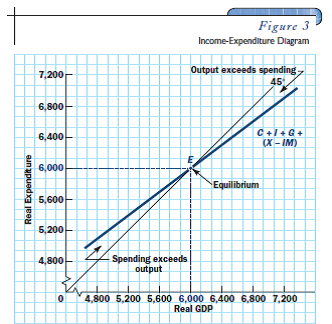Free Essay Answer: Answer: The equilibrium level of GDP is$6,000.
If the real GDP is greater than or less than the equilibrium level, the following problem will occur.
If real GDP is below equilibrium level
If the level of GDP falls to $5,000, then the actual GDP will be less than the planned GDP. When the actual GDP is less than the planned GDP, it will increase the recessionary gap. It implies that the actual GDP is below the potential level. If real GDP is above equilibrium level If the level of GDP increases to$7,000, then the actual GDP will be higher than the planned GDP.
When the actual GDP is greater than the planned GDP, it will increase the inflationary pressure. It implies that the actual GDP is higher than the potential level.

Tags
Choose question tagImagine an economy in which consumer expenditure is represented by the following equation: C = 50 + 0.75 DI Imagine also that investors want to spend $500 at every level of income ( I =$500), net exports are zero ( X - IM = 0), government purchases are $300, and taxes are$200. a. What is the equilibrium level of GDP b. If potential GDP is $3,000, is there a recessionary or inflationary gap If so, how much c. What will happen to the equilibrium level of GDP if investors become optimistic about the country's future and raise their investment to$600 d. After investment has increased to $600, is there a recessionary or inflationary gap How much Essay Answer: Tags Choose question tagReferring to Test Yourself Question 1, do the same for an economy in which investment is$250, net exports are zero, government purchases and taxes are both $400, and the consumption function is as follows: C = 250 + 0.5 DI Reference Test Yourself Question 1, Find the equilibrium level of GDP demanded in an economy in which investment is always$300, net exports are always -$50, the government budget is balanced with purchases and taxes both equal to$400, and the consumption function is described by the following algebraic equation: C = 150 + 0.75 DI ( Hint: Do not forget that DI = Y - T. )
Essay
Tags
Choose question tagDoes the economy this year seem to have an inflationary gap or a recessionary gap (If you do not know the answer from reading the newspaper, ask your instructor.)
Essay
Tags
Choose question tag(More difficult) What would happen to the multiplier analysis if b = 0 If b = 1
Essay
Tags
Choose question tagIn each of these cases, how much saving is there in equilibrium ( Hint: Income not consumed must be saved.) Is saving equal to investment
Essay
Tags
Choose question tagTry to remember where you last spent a dollar. Explain how this dollar will lead to a multiplier chain of increased income and spending. (Who received the dollar What will he or she do with it )
Essay
Tags
Choose question tag(More difficult) 9 Consider an economy in which the consumption function takes the following simple algebraic form: C = 300 + 0.75 DI and in which investment ( I ) is always $900 and net exports are always -$100. Government purchases are fixed at $1,300 and axes are fixed at$1,200. Find the equilibrium level of GDP, and then compare your answer to Table₁ and Figure 2. ( Hint: Remember that disposable income is GDP minus taxes: DI = Y - T = Y - 1,200.)
Essay
Tags
Choose question tag(More difficult) Keep everything the same as in Test Yourself Question 4 except change investment to I = $1,100. Use the equilibrium condition Y = C + I + G + ( X - IM ) to find the equilibrium level of GDP on the demand side. (In working out the answer, assume the price level is fixed.) Compare your answer to Table 3 and Figure₁0. Now compare your answer to the answer to Test Yourself Question 4. What do you learn about the multiplier Table 3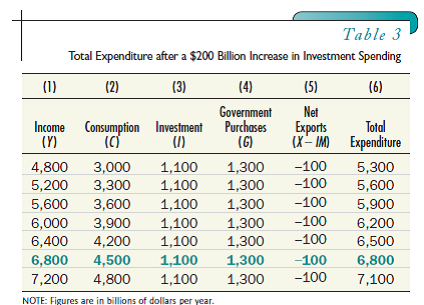Figure₁0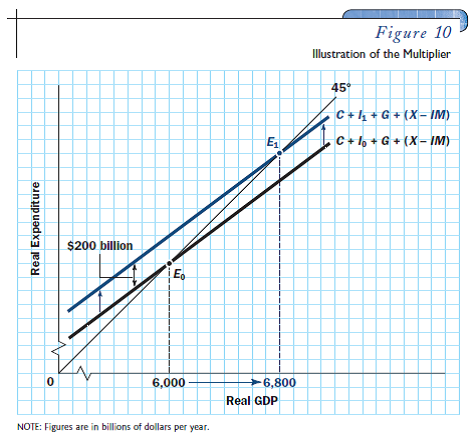Reference Test Yourself Question 4. (More difficult) 9 Consider an economy in which the consumption function takes the following simple algebraic form: C = 300 + 0.75 DI and in which investment ( I ) is always$900 and net exports are always -$100. Government purchases are fixed at$1,300 and axes are fixed at $1,200. Find the equilibrium level of GDP, and then compare your answer to Table₁ and Figure 2. ( Hint: Remember that disposable income is GDP minus taxes: DI = Y - T = Y - 1,200.) Essay Answer: Tags Choose question tagFind the equilibrium level of GDP demanded in an economy in which investment is always$300, net exports are always -$50, the government budget is balanced with purchases and taxes both equal to$400, and the consumption function is described by the following algebraic equation: C = 150 + 0.75 DI ( Hint: Do not forget that DI = Y - T. )
Essay
Tags
Choose question tagFrom the following data, construct an expenditure schedule on a piece of graph paper. Then use the income-expenditure (45° line) diagram to determine the equilibrium level of GDP. Compare your answer with your answer to the previous question.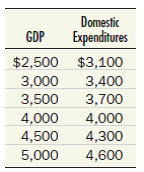Essay
Tags
Choose question tag(More difficult) An economy has the following consumption function: C = 200 + 0.8 DI The government budget is balanced, with government purchases and taxes both fixed at $1,000. Net exports are$100. Investment is $600. Find equilibrium GDP. What is the multiplier for this economy If G rises by$100, what happens to Y What happens to Y if both G and T rise by $100 at the same time Essay Answer: Tags Choose question tagFrom the following data, construct an expenditure schedule on a piece of graph paper. Then use the income-expenditure (45° line) diagram to determine the equilibrium level of GDP.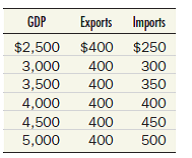Now suppose investment spending rises to$260, and the price level is fixed. By how much will equilibrium GDP increase Derive the answer both numerically and graphically.
Essay
Tags
Choose question tagFredonia has the following consumption function: C = 100 + 0.8 DI Firms in Fredonia always invest $700 and net exports are zero, initially. The government budget is balanced with spending and taxes both equal to$500. a. Find the equilibrium level of GDP. b. How much is saved Is saving equal to investment c. Now suppose that an export-promotion drive succeeds in raising net exports to $100. Answer (a) and (b) under these new circumstances. Essay Answer: Tags Choose question tagIf domestic expenditure (the sum of C + I + G in the economy described in Test Yourself Question 1) is as shown in the following table, construct a 45° line diagram and locate the equilibrium level of GDP.Essay Answer: Tags Choose question tagUse both numerical and graphical methods to find the multiplier effect of the following shift in the consumption function in an economy in which investment is always$220, government purchases are always $100, and net exports are always -$40. (Hint: What is the marginal propensity to consume )Essay
Tags
Choose question tagSuppose exports and imports of a country are given by the following:Calculate net exports at each level of GDP.
EssaySuppose that investment spending is always $250, government purchases are$100, net exports are always \$50, and consumer spending depends on the price level in the following way: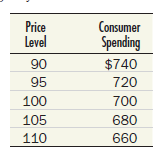On a piece of graph paper, use these data to construct an aggregate demand curve. Why do you think this example supposes that consumption declines as the price level rises